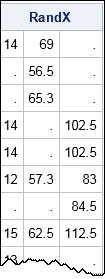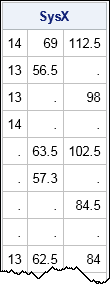When simulating data or testing algorithms, it is useful to be able to generate patterns of missing data. This article shows how to generate random and systematic patterns of missing values. In other words, this article shows how to replace nonmissing data with missing data.

### Generate a random pattern of missing values

The following SAS/IML program reads numerical data into a matrix from the Sashelp.Class data set. The matrix has 16 rows and three columns. The program then generates a matrix of the same size that contains a random pattern of zeros and ones, where about 40% of the values will be ones. The LOC function is used to find the locations of the ones, and the corresponding locations in the data are set to missing:

```proc iml; use Sashelp.Class; /* read numeric data into X */ read all var _NUM_ into X; close;   /* random assignment of missing values */ RandX = X; /* copy data */ p = 0.4; /* approx proportion of missing elements */ call randseed(1234); B = randfun(dimension(X), "bern", p); /* random 0s or 1s */ missIdx = loc(B=1); /* find position of 1s */ if ncol(missIdx)>0 then RandX[missIdx] = .; /* replace 1s with missing */ print RandX;```In this way, you can replace a certain percentage of the data values with missing values.

### Generate a systematic pattern of missing values

In the preceding section, the technique for inserting missing values does not use the fact that the matrix B is random. The technique works with any zero-one matrix B that specifies a pattern of missing values. For example, you can create a matrix that contains all combinations of zeros and ones, then use that pattern to set missing values, as follows:

```C = { 0 0 0, 0 0 1, 0 1 0, 0 1 1, 1 0 0, 1 0 1, 1 1 0, 1 1 1 }; /* pattern matrix */   missIdx = loc(C=1); SysX = X; /* copy data */ if ncol(missIdx)>0 then SysX[missIdx] = .; /* replace 1s with missing */ print SysX;```You could also specify the locations of the missing values by using subscripts of the data matrix. You can use the SUB2NDX function to convert subscripts to indices.

### Patterns of missing data by using the SAS DATA step

In the SAS DATA step you can use arrays to create a random pattern of missing values. For example, the following SAS data set reads numerical variables from the Sashelp.Class data and randomly assigns 40% of the data to missing values:

```/* generate missing values in random locations */ data RandClass(drop=i); call streaminit(1234); set Sashelp.Class(keep=_NUMERIC_); array x {*} _numeric_; do i = 1 to dim(x); if rand("Bern", 0.4) then /* p=0.4 ==> about 40% missing */ x[i]=.; end; run;   proc print; run;```

The output is not shown, but the random pattern is identical to the random pattern that was generated by using SAS/IML matrices.

You could use the DATA step to specify patterns of missing values for which there is a formula, such as every fourth data value (MOD(cnt,4)=1). However, it is less easy to generate an arbitrary pattern, such as the "all combinations" pattern in the previous section. In general, I think the SAS/IML approach is easier to use and more flexible.

For any pattern of missing values, you can use PROC MI to summarize the pattern. You can also use various graphical techniques to visualize the pattern of missing data.

Share